The Essential R Reference Book

Our book The Essential R Reference can help you learn R by helping you find the R functions you need to carry out a range of tasks in data analysis, data visualisation and much more. The Essential R Reference is a topic-led R reference book, a cross between a dictionary, glossary and thesaurus for R, using plenty of practical examples.

Essential R is an R reference book that can help you learn data science, and find the tools you need to be using R for data analytics. Essential R is topic-led R reference book; you look for the topic you want and find the R functions you need. Each R function is presented with the essential parameters, making it easier to follow. There are also examples of each R command “in action” so you can gain a better understanding of how the R function works.

Book Overview - Essential R Reference

The Essential R Reference is a book about data analytics with R and using R for data science. Essential R is an R reference book with a topic-led approach; you look up the topic you want and find the R functions you need. If you are learning about using R for data science, then this approach can help you find what you need.

The Essential R reference book helps you learn R by being a dictionary, glossary and thesaurus for R, with plenty of practical examples. Essential R is an R reference book that can help with R data analysis, with plenty of examples of how to use R functions for a range of data analysis and data visualisation tasks.

If you are learning R and need a data analysis with R book, then Essential R can help you find the R functions you need. Each R function is listed with other related R commands, so you can learn R more completely by finding new ways to accomplish the R data analytics tasks you need.

Essentials Covered in this Book

If you are learning R and need a data analysis with R book, The Essential R Reference can help you find the R functions you need. Essential R is a different kind of R book; learn R essentials in a topic-led manner; look up the topic and find the R commands you need.

Use Essential R to help you learn data analytics with R; each function is shown with examples and presented with the “essential” parameters and examples, making it easier to follow.

The Essential R reference is an R book that covers R essentials grouped in various themes:

• Math and Statistics.

Use The Essential R Reference to help you learn R for data science by providing you with a topic-led reference. This R book will help you with the R essentials needed for conducting R data analysis and data visualisation. Key highlights include:

• Topic-based help for using R
• Examples of usage for R commands
• Examples for R maths and statistics functions
• Examples for R data functions
• Examples for R graphics functions

Essential R can help novice users learn more easily and provide more experienced users with a reference work they can delve into time and time again.

My Publications

I have written several books on ecology and data analysis

Statistics for Ecologists
Using R and Excel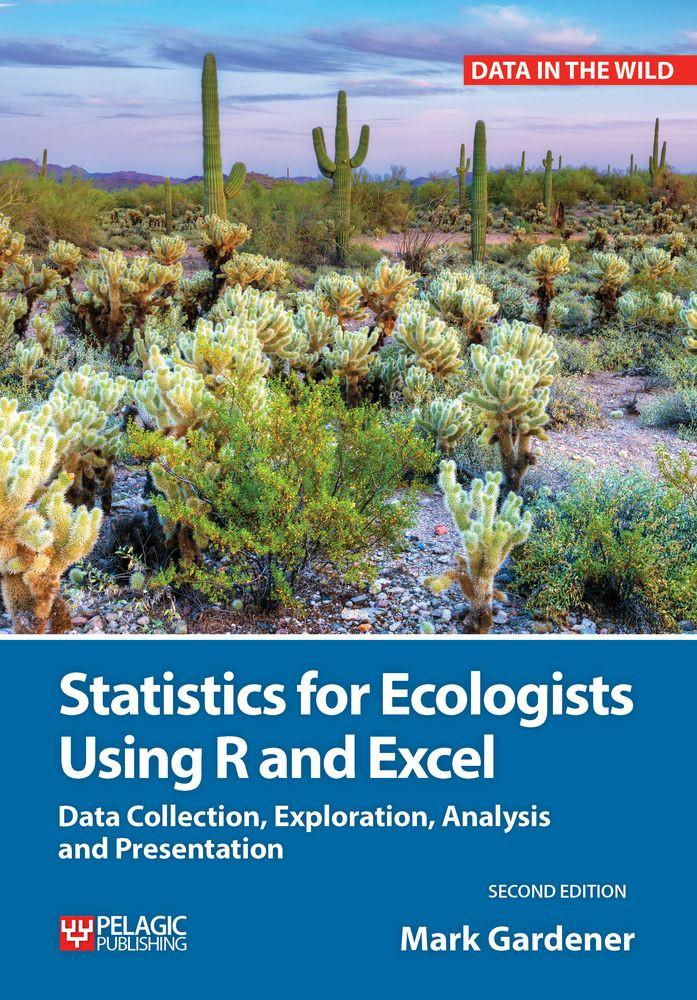£34.99
Beginning R: The Statistical
Programming Language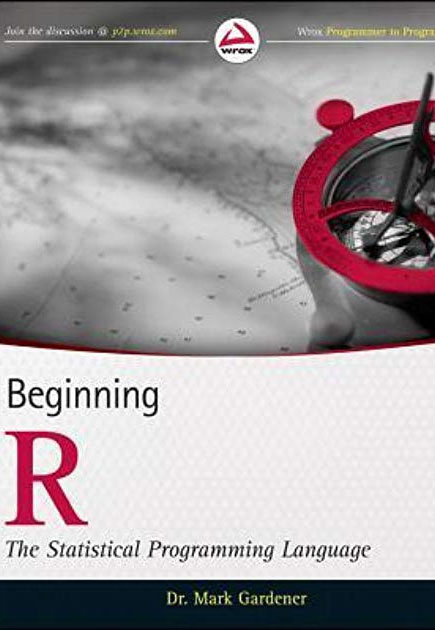£26.99
The Essential R
Reference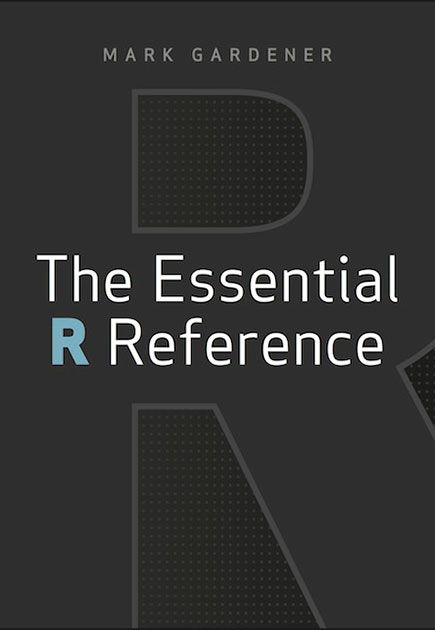£44.99
Community
Ecology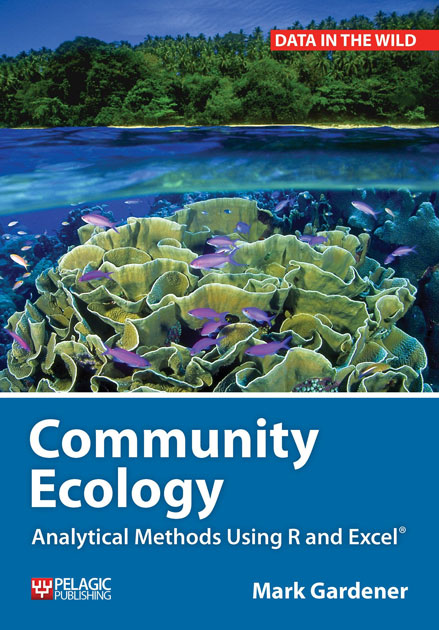£39.99
Managing Data
Using Excel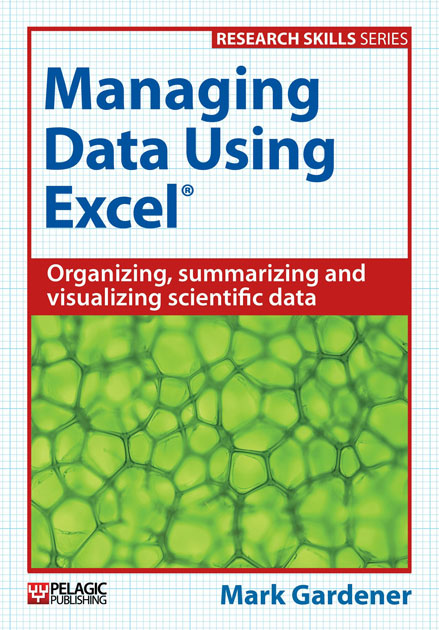£24.99

Register your interest for our Training Courses

We run training courses in data management, visualisation and analysis using Excel and R: The Statistical Programming Environment. Courses will be held at one of our training centres in London. Alternatively we can come to you and provide the training at your workplace. Training Courses are also available via an online platform.

Get In Touch Now

for any information regarding our training courses, publications or help with a data project